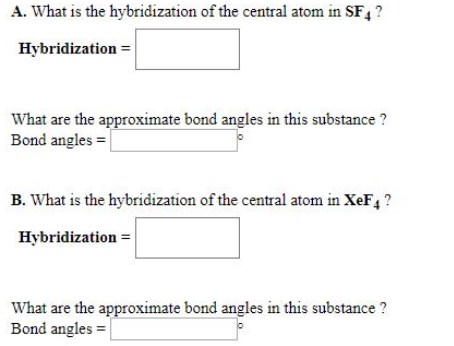# Problem: A. What is the hybridization of the central atom in SF4? What are the approximate bond angles in this substance? B. What is the hybridization of the central atom in XeF4? What are the approximate bond angles in this substance?

###### FREE Expert Solution
98% (374 ratings)###### Problem Details

A. What is the hybridization of the central atom in SF4

What are the approximate bond angles in this substance?

B. What is the hybridization of the central atom in XeF4

What are the approximate bond angles in this substance?